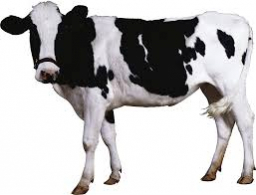# Cows and pigs

There are 3/4 as many cows as pigs on an animal farm. If there are 35 pigs and cows on the farm, how many of them are pigs?

p =  20

### Step-by-step explanation:Did you find an error or inaccuracy? Feel free to write us. Thank you!

Tips for related online calculators
Need help calculating sum, simplifying, or multiplying fractions? Try our fraction calculator.
Do you have a linear equation or system of equations and looking for its solution? Or do you have a quadratic equation?